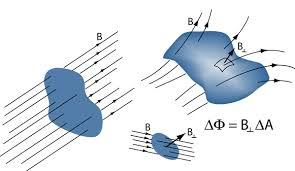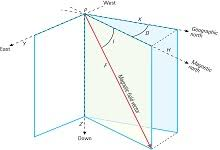## How to Calculate and Solve for Magnetic Flux | Magnetic FieldThe image above represents magnetic flux.

To compute for magnetic flux, two essential parameters are needed and these parameters are Area (A) and Total Magnetic Field (B).

The formula for calculating magnetic flux:

φ = B.A

Where:

φ = Magnetic Flux
A = Area
B = Total Magnetic Field

Let’s solve an example;
Find the magnetic flux with a given area of 32 and a total magnetic field of 18.

This implies that;

A = Area = 32
B = Total Magnetic Field = 18

φ = B.A
φ = (18)(32)
φ = 576

Therefore, the magnetic flux is 576 Wb.

Calculating the Area when the Magnetic Flux and the Total Magnetic Field is Given.

A = φ / B

Where;

A = Area
φ = Magnetic Flux
B = Total Magnetic Field

Let’s solve an example;
Find the area when the magnetic flux is 40 and the total magnetic field is 20.

This implies that;

φ = Magnetic Flux = 40
B = Total Magnetic Field = 20

A = φ / B
A = 40 / 20
A = 2

Therefore, the area is 2.

## How to Calculate and Solve for Total Magnetic Field (H, J) | Magnetic FieldThe image above represents total magnetic field.

To compute for total magentic field, three essential parameters are needed and these parameters are Magnetic Permeability of Vacuum (μo), Magnetizing Force of Inducing Field (H) and Intensity of Induced Magnetization (J).

The formula for calculating total magnetic field:

B = μoH + μoJ

Where:

B = Total Magnetic Field
μo = Magnetic Permeability of Vacuum
H = Magnetizing Force of Inducing Field
J = Intensity of Induced Magnetization

Let’s solve an example;
Find the total magnetic field when the magnetic permeability of vaccum is 1.2566371E-6, magnetizing force of inducing field is 34 and the intensity induced magnetization is 28.

This implies that;

μo = Magnetic Permeability of Vacuum = 1.2566371E-6
H = Magnetizing Force of Inducing Field = 34
J = Intensity of Induced Magnetization = 28

B = μoH + μoJ
B = (0.0000012566371)(34) + (0.0000012566371)(28)
B = 0.0000427256614 + 0.0000351858388
B = 0.0000779115002

Therefore, the total magnetic field is 0.0000779115002 T.